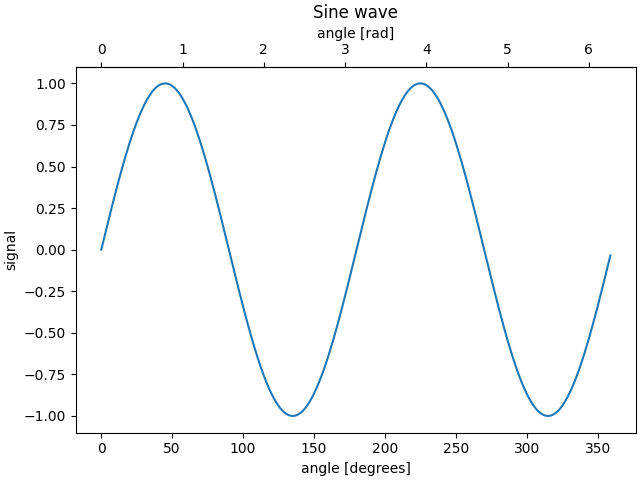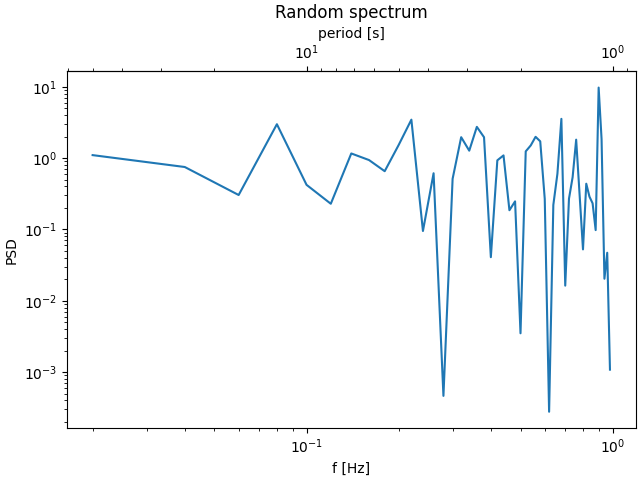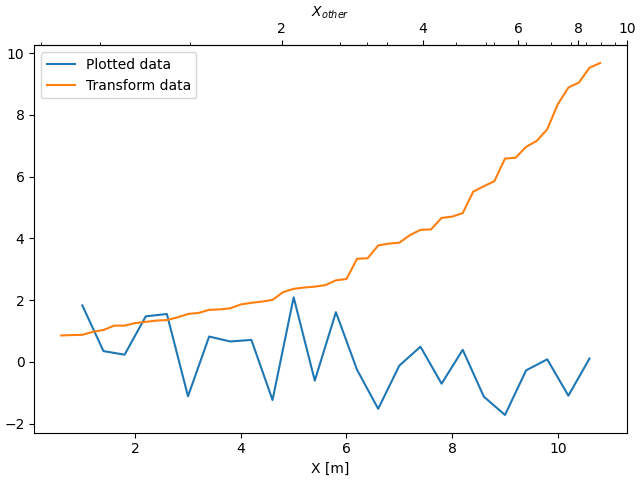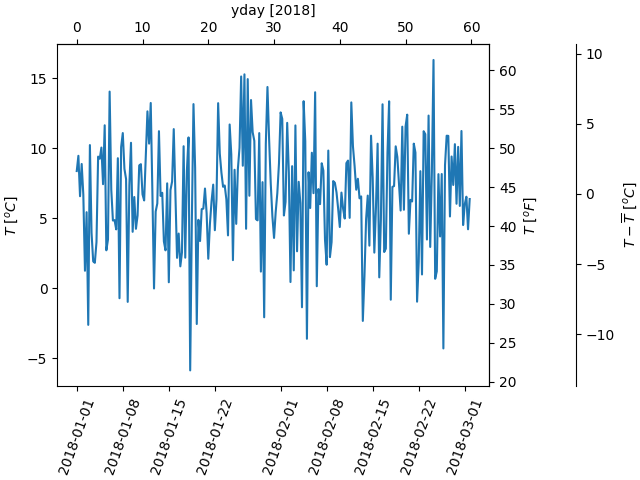# Secondary Axis¶

Sometimes we want a secondary axis on a plot, for instance to convert radians to degrees on the same plot. We can do this by making a child axes with only one axis visible via axes.Axes.secondary_xaxis and axes.Axes.secondary_yaxis. This secondary axis can have a different scale than the main axis by providing both a forward and an inverse conversion function in a tuple to the functions kwarg:

import matplotlib.pyplot as plt
import numpy as np
import datetime
import matplotlib.dates as mdates
from matplotlib.ticker import AutoMinorLocator

fig, ax = plt.subplots(constrained_layout=True)
x = np.arange(0, 360, 1)
y = np.sin(2 * x * np.pi / 180)
ax.plot(x, y)
ax.set_xlabel('angle [degrees]')
ax.set_ylabel('signal')
ax.set_title('Sine wave')

return x * np.pi / 180

return x * 180 / np.pi

plt.show()Here is the case of converting from wavenumber to wavelength in a log-log scale.

Note

In this case, the xscale of the parent is logarithmic, so the child is made logarithmic as well.

fig, ax = plt.subplots(constrained_layout=True)
x = np.arange(0.02, 1, 0.02)
np.random.seed(19680801)
y = np.random.randn(len(x)) ** 2
ax.loglog(x, y)
ax.set_xlabel('f [Hz]')
ax.set_ylabel('PSD')
ax.set_title('Random spectrum')

def one_over(x):
"""Vectorized 1/x, treating x==0 manually"""
x = np.array(x).astype(float)
near_zero = np.isclose(x, 0)
x[near_zero] = np.inf
x[~near_zero] = 1 / x[~near_zero]
return x

# the function "1/x" is its own inverse
inverse = one_over

secax = ax.secondary_xaxis('top', functions=(one_over, inverse))
secax.set_xlabel('period [s]')
plt.show()Sometime we want to relate the axes in a transform that is ad-hoc from the data, and is derived empirically. In that case we can set the forward and inverse transforms functions to be linear interpolations from the one data set to the other.

Note

In order to properly handle the data margins, the mapping functions (forward and inverse in this example) need to be defined beyond the nominal plot limits.

In the specific case of the numpy linear interpolation, numpy.interp, this condition can be arbitrarily enforced by providing optional kwargs left, right such that values outside the data range are mapped well outside the plot limits.

fig, ax = plt.subplots(constrained_layout=True)
xdata = np.arange(1, 11, 0.4)
ydata = np.random.randn(len(xdata))
ax.plot(xdata, ydata, label='Plotted data')

xold = np.arange(0, 11, 0.2)
# fake data set relating x coordinate to another data-derived coordinate.
# xnew must be monotonic, so we sort...
xnew = np.sort(10 * np.exp(-xold / 4) + np.random.randn(len(xold)) / 3)

ax.plot(xold[3:], xnew[3:], label='Transform data')
ax.set_xlabel('X [m]')
ax.legend()

def forward(x):
return np.interp(x, xold, xnew)

def inverse(x):
return np.interp(x, xnew, xold)

secax = ax.secondary_xaxis('top', functions=(forward, inverse))
secax.xaxis.set_minor_locator(AutoMinorLocator())
secax.set_xlabel('$X_{other}$')

plt.show()A final example translates np.datetime64 to yearday on the x axis and from Celsius to Fahrenheit on the y axis. Note the addition of a third y axis, and that it can be placed using a float for the location argument

dates = [datetime.datetime(2018, 1, 1) + datetime.timedelta(hours=k * 6)
for k in range(240)]
temperature = np.random.randn(len(dates)) * 4 + 6.7
fig, ax = plt.subplots(constrained_layout=True)

ax.plot(dates, temperature)
ax.set_ylabel(r'$T\ [^oC]$')
plt.xticks(rotation=70)

def date2yday(x):
"""Convert matplotlib datenum to days since 2018-01-01."""
y = x - mdates.date2num(datetime.datetime(2018, 1, 1))
return y

def yday2date(x):
"""Return a matplotlib datenum for *x* days after 2018-01-01."""
y = x + mdates.date2num(datetime.datetime(2018, 1, 1))
return y

secax_x = ax.secondary_xaxis('top', functions=(date2yday, yday2date))
secax_x.set_xlabel('yday ')

def celsius_to_fahrenheit(x):
return x * 1.8 + 32

def fahrenheit_to_celsius(x):
return (x - 32) / 1.8

secax_y = ax.secondary_yaxis(
'right', functions=(celsius_to_fahrenheit, fahrenheit_to_celsius))
secax_y.set_ylabel(r'$T\ [^oF]$')

def celsius_to_anomaly(x):
return (x - np.mean(temperature))

def anomaly_to_celsius(x):
return (x + np.mean(temperature))

# use of a float for the position:
secax_y2 = ax.secondary_yaxis(
1.2, functions=(celsius_to_anomaly, anomaly_to_celsius))
secax_y2.set_ylabel(r'$T - \overline{T}\ [^oC]$')

plt.show()References

The use of the following functions, methods, classes and modules is shown in this example:

Total running time of the script: ( 0 minutes 3.327 seconds)

Keywords: matplotlib code example, codex, python plot, pyplot Gallery generated by Sphinx-Gallery